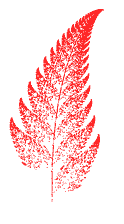Home > General Resources > Recent Talks > Iterated Functions

# Iterated Functions in Precalculus: Doing It Again and Again with Sketchpad

NCTM 2005
Scott Steketee, Key Curriculum Press

 The sketches and the student sheets for many of the activities used in this presentation are available to the right.The teacher notes for these activities, and approximately 50 additional precalculus activities using Sketchpad, are contained in Exploring Precalculus with The Geometer's Sketchpad, published by Key Curriculum Press.There are also three activities to provide users with step-by-step instructions for creating various iterations from scratch. You can use these activities to learn how to do iteration in Sketchpad as you create a spiral design, a Fibonacci series, and an Euler's-method approximation of the anti-derivative.Iteration in Mathematics

There are several big ideas in mathematics that form unifying themes across different branches of mathematics, including functions, transformations, proof, and data. Iteration is also such a topic, with important applications throughout the curriculum. Understanding iteration, the way in which systematically repeated simple operations can build complex structures, can shed light on many important concepts in arithmetic, algebra, geometry, fractals, calculus, and mathematical modeling.

Iteration is also at the heart of the distinction between discrete and continuous processes. This distinction begins with the concept of number and comes into play throughout mathematics. Even the process of graphing a continuous function (whether it's done by hand, by graphing calculator, or by Sketchpad), is accomplished in practice by plotting a discrete set of points and interpolating between data points. This interplay is fundamental to mathematics: The definition of continuity itself depends on the concept of a limit, involving ever-smaller discrete intervals.

Because iteration allows us to move from the discrete to the continuous, it is a powerful tool for elucidating many mathematical topics, ranging from the counting numbers to calculus.

Iteration and Technology

Iteration often involves large numbers of operations that would be difficult and time-consuming to carry out by hand, so it's particularly suited to the use of technology. The value of graphing calculators lies in their ability to perform the iterations required to graph and manipulate functions. Similarly, Sketchpad makes it possible, by means of iteration, to investigate mathematical phenomena that would be computationally intractable without technology.

Iteration in Precalculus

In addition to the many topics where iteration is an implicit part of the mathematics studied in precalculus, there are a number in which iteration plays a more explicit role. We will look at a few of those today, using prepared student activities and sketches.

Materials

The downloadable sketches and student sheets contain the following activities:

• Generating Arithmetic/Geometric Sequences Numerically—Students develop an understanding of arithmetic and geometric sequences by building and modifying them with Sketchpad.
• A Sequence Approach to Logs—In this activity students graph geometric sequences against arithmetic sequences to obtain good approximations of log curves.
• Compound Interest—In this activity students use iterated calculations to compute and plot the value of a compound interest investment. This is connected to the constant e and the general formula for continuous compounding.
• A Geometric Approach to e—Students use a limit definition of e, along with multiplication on the complex plane, to find the value of e.
• The Logistic Function—In this activity students build a Sketchpad model of a population for which the growth is restrained by some factor. In the process, students explore the sensitivity of the long-term behavior to the initial size of the population and to the parameters that determine the growth.
• The Taylor Series—In this activity students explore how adding terms to a Taylor series approximation increases the accuracy of the approximation.
• The Sierpinski Gasket—In this activity students create various fractal designs (including the Sierpinski triangle and Mira, Julia, and Mandelbrot fractals) as strange attractors.
• Barnsley's Fern—Students plot points using four pairs of iterated functions. By choosing randomly among the function pairs, they create a fractal Barnsley's Fern.